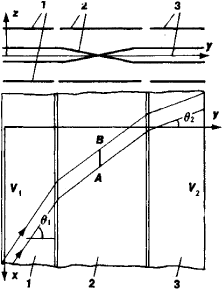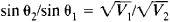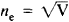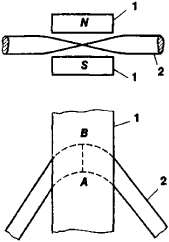# Electron Prism

The following article is from The Great Soviet Encyclopedia (1979). It might be outdated or ideologically biased.

## Electron Prism

Electron-optical or ion-optical systems that are used to deflect charged particle beams or to separate charged particles by energy or mass are called electron prisms or ion prisms, respectively. The term “electron prism” was adopted in the framework of the general analogy between electron and ion optics and the optics of light rays.

Among the numerous types of electron prisms, the closest analogs to optical prisms are those in which a parallel beam ofFigure 1. A telescopic system consisting of two cylindrical immersion electrostatic lenses: (1) and (2) electrodes constituting the first cylindrical lens in the path of a beam, (2) and (3) second lens; (AB) focal line. The bent lines with the arrows represent the projections of the charged particle trajectories on the yz and xy planes. (As applied to electron lenses, the term “cylindrical” indicates that such lenses can act on an electron beam in the same way as a cylindrical optical lens acts on a light beam.)

charged particles that enters a prism remains parallel after being deflected. The simplest electrostatic prism that preserves beam parallelism is a telescopic system consisting of two cylindrical immersion electron lenses (Figure 1). The rear focal line AB of the first lens coincides with the front focal line of the second lens. The electrostatic field of a telescopic system is “two-dimensional”—that is, does not vary in the direction parallel to the x-axis—and is symmetric with respect to the midplane xy, near which the particles move.

A parallel beam enters a telescopic system at a large angle of θ1 to the y-axis and exits at an angle of θ2, preserving its parallelism. In this case, the following equation is satisfied:where V1 is the potential of the first section of the prism and of the region of space in front of that section and V2 is the potential of the last section and of the region of space behind that section.

An electrostatic potential can be determined with an accuracy up to an arbitrary constant and is assumed to be equal to zero when required by considerations of convenience. In the case of a telescopic electrostatic prism, as in most problems of electron and ion optics, the potential is assumed to be equal to zero when the particle velocity is equal to zero. Under this condition, the electron-optical refractive index. Thus, the deflection of a charged particle beam in a telescopic system obeys a law that is completely analogous to Snell’s law in light optics.Figure 2. The deflection of a charged particle beam by a magnetic prism: (1) magnetic poles of the prism, (2) charged particle beam; (AB) local line

To increase dispersion, compound electron prisms are used. Such prisms consist of two telescopic systems at an angle to one another. Compound electron prisms are used as dispersing elements in electron spectrometers.

In magnetic prisms with a “two-dimensional” field, the role of cylindrical lenses is played by scattering fields at the edges of the magnetic poles. At a certain angle of beam incidence on a prism, the scattering fields form a telescopic system (Figure 2).

### REFERENCES

Artsimovich, L. A., and S. Iu. Luk’ianov. Dvizhenie zariazhennykh chastits v elektricheskikh i magnitnykh poliakh. Moscow, 1972.
Kel’man, V. M., and S. Ia. Iavor. Elektronnaia optika, 3rd ed. Leningrad, 1968.
Prizmennye beta-spektrometry i ikh primenenie. Vilnius, 1971.
Primenenie prizmennykh beta-spektrometrov. Vilnius, 1974.

V. M. KEL’MAN and I. V. RODNIKOVA